IntroductionIntroduction# Unit Conversion

INTRO
A unit conversion takes the value of a physical property (time, length, mass/weight, volume, etc.) and expresses it using a different unit of measurement.

For example, we can convert to . Even though these two measurements look different, they actually have the same value.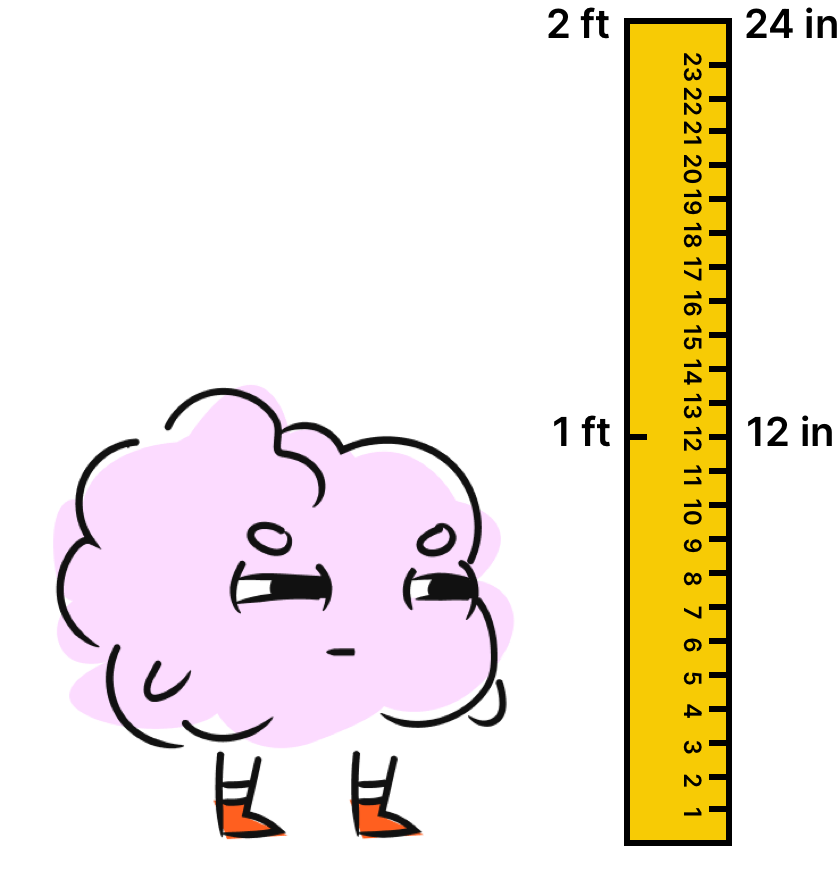This is similar to how we can express our thoughts and feelings using emojis in place of words.

Instead of typing, “but that’s none of my business,” we can convert the words to emojis, “🐸☕,” and call it a day. The expression changes, but the meaning (or value) stays the same.To convert units, we use a process called dimensional analysis.

CALCULATOR

## Unit Conversion Calculator

What type of measurement do you want to convert?

KEY STEPS

## How to Convert Units

### Step 1. Identify the given unit (starting position) and the unknown unit (ending position).

The given unit is the unit that has a given number.
The unknown unit is the unit we need to find the unknown number of.

### Step 2. Identify the path from start to finish.

Select the set of conversion factors that can get us from the given unit to the unknown unit.

### Step 3. Use the conversion factors to set up an equation that cancels out unwanted units and gets the unknown unit by itself.

To cancel out, we need a matching top half for every bottom half.

LESSON
Converting Units

## Converting Units

When we convert a unit, we’re just finding another way to express the same value using a different unit of measurement.

To convert a unit, we use dimensional analysis, which involves multiplying the original measurement by a pathway of conversion factors that gets us from the given unit to the unknown unit.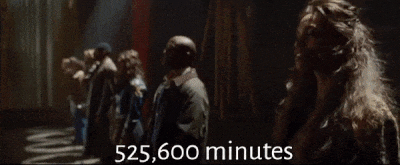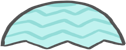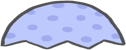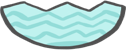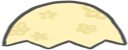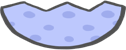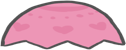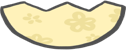We can cancel out unwanted units as long as they show up an equal number of times on the top and bottom.

Think of this as needing a matching bottom half for every top half to close an Easter egg and cancel out.

Let’s walk through some step-by-step examples of how to convert units!

PRACTICE
Converting Units

## Practice: Converting Units

Question 1 of 10: Convert 1 hours to minutes.

### Step 1. Identify the given unit (starting position) and the unknown unit (ending position).

The given unit is the unit that has a given number.
The unknown unit is the unit we need to find the unknown number of.
Given:
Unknown:

CONCLUSION
Sheesh, look at you go! Thanks for checking out this lesson ☺️🙏. Where to next?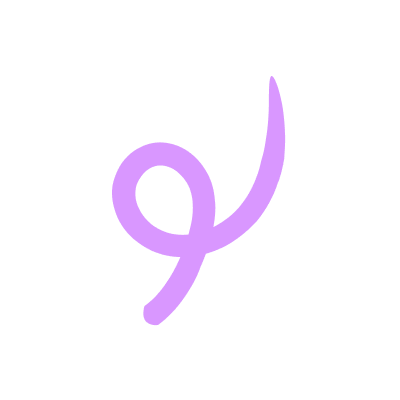Leave Feedback